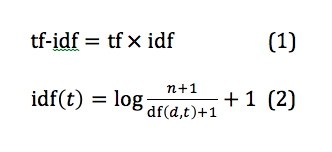# Global AI and Data Science

View Only

## Similarity Measure between Two Documents

####By Moloy De posted Thu June 18, 2020 08:36 PMThe cosine similarity is the cosine of the angle between two vectors. Figure above shows three 3-dimensional vectors and the angles between each pair. In text analysis, each vector can represent a document. The greater the value of θ, the less the value of cos θ, thus the less the similarity between two documents.Raw texts are pre-processed with the most common words and punctuation removed, tokenization, and stemming or lemmatization.

A dictionary of unique terms found in the whole corpus is created. Texts are quantified first by calculating the term frequency (tf) for each document. The numbers are used to create a vector for each document where each component in the vector stands for the term frequency in that document. Let n be the number of documents and m be the number of unique terms. Then we have an n by m tf matrix.

The core of the rest is to obtain a “term frequency-inverse document frequency” (tf-idf) matrix. Inverse document frequency is an adjustment to term frequency. This adjustment deals with the problem that certain terms do occur more than others. Thus, tf-idf scales up the importance of rarer terms and scales down the importance of more frequent terms relative to the whole corpus.

The idea of the weighting effect of tf-idf is better expressed in the two equations below (the formula for idf is the default one used by scikit-learn : the 1 added to the denominator prevents division by 0, the 1 added to the nominator makes sure the value of the ratio is greater than or equal to 1, the third 1 added makes sure that idf is greater than 0, i.e., for an extremely common term t for which n = df(d,t), its idf is at least not 0 so that its tf still matters; Note that there is only one 1 added to the denominator, which results in negative values after taking the logarithm for some cases. Negative value is difficult to interpret.where n is the total number of documents and df(d, t) is the number of documents in which term t appears. In Equation 2, as df(d, t) gets smaller, idf(t) gets larger. In Equation 1, tf is a local parameter for individual documents, whereas idf is a global parameter taking the whole corpus into account.

Therefore, even the tf for one term is very high for document d1, if it appears frequently in other documents (with a smaller idf), its importance of “defining” d1 is scaled down. On the other hand, if a term has high tf in d1 and does not appear in other documents (with a greater idf), it becomes an important feature that distinguishes d1 from other documents.

The calculated tf-idf is normalized by the Euclidean norm so that each row vector has a length of 1. The normalized tf-idf matrix should be in the shape of n by m. A cosine similarity matrix (n by n) can be obtained by multiplying the if-idf matrix by its transpose (m by n).

I downloaded three PDF’s of Quran, Bible and Gita. Below are the similarity measures.REFERENCE : Measuring Similarity Between Texts in Python

#GlobalAIandDataScience
#GlobalDataScience
#Hands-on
#Highlights
#Highlights-home